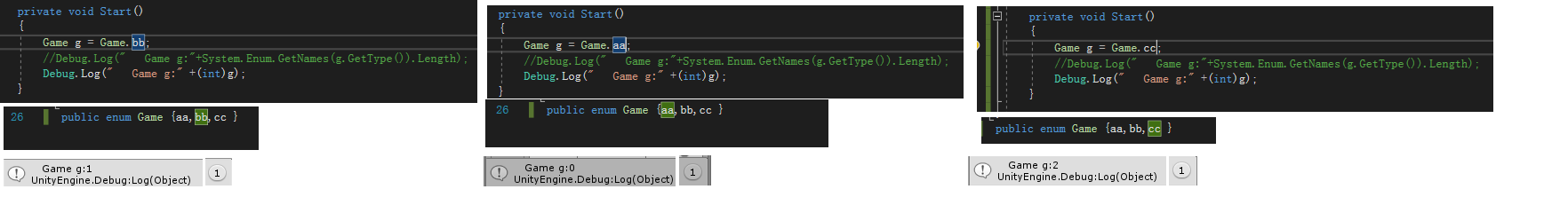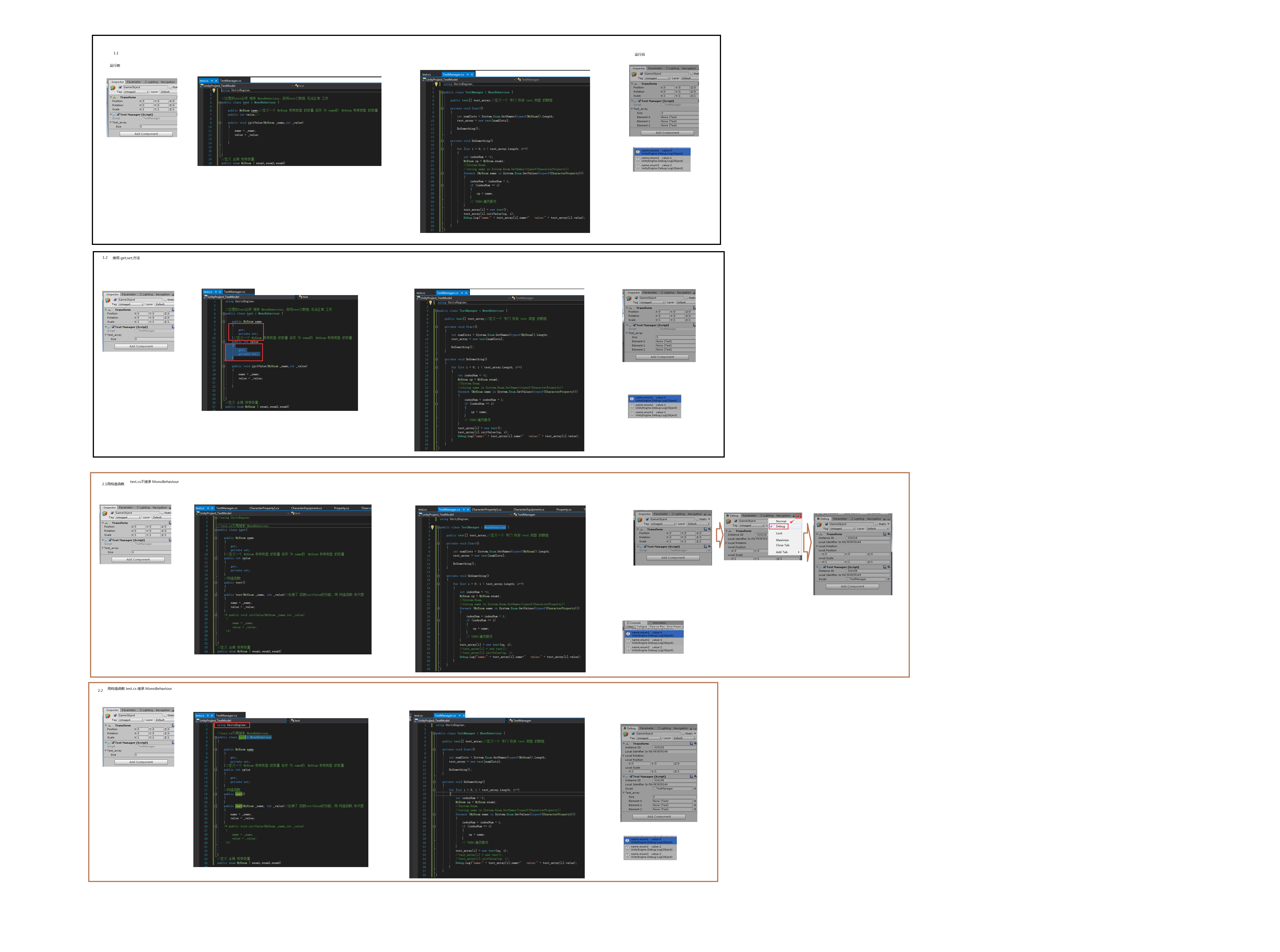[Unity]枚举类型变量对应的序号和名字。get;set;方法，构造函数的使用`Game g = Game.cc;        //Debug.Log("   Game g:"+System.Enum.GetNames(g.GetType()).Length);        Debug.Log("   Game g:" +(int)g);`
`public enum Game {aa,bb,cc }`

-----------------------------以下都是废话1.1常规方法实现 显示枚举类型变量 对应的序号和名字

test.cs

`using UnityEngine;//这里的test必须 继承 MonoBehaviour，否则test[]数组 无法正常 工作public class test : MonoBehaviour {    public MyEnum name;//定义一个 MyEnum 枚举类型 的变量 名字 为 name的  MyEnum 枚举类型 的变量    public int value;//    public void initValue(MyEnum _name,int _value)    {        name = _name;        value = _value;    }}//定义 全局 枚举变量public enum MyEnum { enum1,enum2,enum3}`

TestManager.cs

`using UnityEngine;public class TestManager : MonoBehaviour {    public test[] test_array;//定义一个 专门 存放 test 类型 的数组    private void Start()    {        int numSlots = System.Enum.GetNames(typeof(MyEnum)).Length;        test_array = new test[numSlots];        DoSomething();    }    private void DoSomething()    {        for (int i = 0; i < test_array.Length; i++)        {            int indexNum = -1;            MyEnum cp = MyEnum.enum1;            //System.Enum.            //string name in System.Enum.GetNames(typeof(CharacterProperty))            foreach (MyEnum name in System.Enum.GetValues(typeof(CharacterProperty)))            {                indexNum = indexNum + 1;                if (indexNum == i)                {                    cp = name;                }                // TODO:遍历操作                }            test_array[i] = new test();            test_array[i].initValue(cp, i);            Debug.Log("name:" + test_array[i].name+"   value:" + test_array[i].value);        }    }}`

1.2使用 get;set;方法

get;set;方法限制 外部，其他的 脚本 随意 的 set设置 test.cs 的内部的变量，起到保护的作用，另一方面

test.cs改动

`using UnityEngine;//这里的test必须 继承 MonoBehaviour，否则test[]数组 无法正常 工作public class test : MonoBehaviour {    public MyEnum name    {        get;        private set;    }//定义一个 MyEnum 枚举类型 的变量 名字 为 name的  MyEnum 枚举类型 的变量    public int value    {        get;        private set;    }    public void initValue(MyEnum _name,int _value)    {        name = _name;        value = _value;    }}//定义 全局 枚举变量public enum MyEnum { enum1,enum2,enum3}`

TestManager.cs没有改动

2.1使用 构造函数 来代替initValue函数

test.cs

`//using UnityEngine;//test.cs不再继承 MonoBehaviourpublic class test {    public MyEnum name    {        get;        private set;    }//定义一个 MyEnum 枚举类型 的变量 名字 为 name的  MyEnum 枚举类型 的变量    public int value    {        get;        private set;    }    //构造函数    public test()    {    }    public test(MyEnum _name, int _value)//去掉了 函数initValue的功能，用 构造函数 来代替    {        name = _name;        value = _value;    }    /* public void initValue(MyEnum _name,int _value)     {         name = _name;         value = _value;     }*/}//定义 全局 枚举变量public enum MyEnum { enum1,enum2,enum3}`

TestManager.cs

`using UnityEngine;public class TestManager : MonoBehaviour {    public test[] test_array;//定义一个 专门 存放 test 类型 的数组    private void Start()    {        int numSlots = System.Enum.GetNames(typeof(MyEnum)).Length;        test_array = new test[numSlots];        DoSomething();    }    private void DoSomething()    {        for (int i = 0; i < test_array.Length; i++)        {            int indexNum = -1;            MyEnum cp = MyEnum.enum1;            //System.Enum.            //string name in System.Enum.GetNames(typeof(CharacterProperty))            foreach (MyEnum name in System.Enum.GetValues(typeof(CharacterProperty)))            {                indexNum = indexNum + 1;                if (indexNum == i)                {                    cp = name;                }                // TODO:遍历操作                }            test_array[i] = new test(cp, i);            //test_array[i] = new test();            //test_array[i].initValue(cp, i);            Debug.Log("name:" + test_array[i].name+"   value:" + test_array[i].value);        }    }}`

2.2通过试验观察test.cs 是否继承MonoBehaviour 的影响

test.cs

`using UnityEngine;//test.cs不再继承 MonoBehaviourpublic class test : MonoBehaviour{    public MyEnum name    {        get;        private set;    }//定义一个 MyEnum 枚举类型 的变量 名字 为 name的  MyEnum 枚举类型 的变量    public int value    {        get;        private set;    }    //构造函数    public test()    {    }    public test(MyEnum _name, int _value)//去掉了 函数initValue的功能，用 构造函数 来代替    {        name = _name;        value = _value;    }    /* public void initValue(MyEnum _name,int _value)     {         name = _name;         value = _value;     }*/}//定义 全局 枚举变量public enum MyEnum { enum1,enum2,enum3}`
TestManager.cs
`using UnityEngine;public class TestManager : MonoBehaviour {    public test[] test_array;//定义一个 专门 存放 test 类型 的数组    private void Start()    {        int numSlots = System.Enum.GetNames(typeof(MyEnum)).Length;        test_array = new test[numSlots];        DoSomething();    }    private void DoSomething()    {        for (int i = 0; i < test_array.Length; i++)        {            int indexNum = -1;            MyEnum cp = MyEnum.enum1;            //System.Enum.            //string name in System.Enum.GetNames(typeof(CharacterProperty))            foreach (MyEnum name in System.Enum.GetValues(typeof(CharacterProperty)))            {                indexNum = indexNum + 1;                if (indexNum == i)                {                    cp = name;                }                // TODO:遍历操作                }            test_array[i] = new test(cp, i);            //test_array[i] = new test();            //test_array[i].initValue(cp, i);            Debug.Log("name:" + test_array[i].name+"   value:" + test_array[i].value);        }    }}`

.cs脚本 继承 MonoBehaviour1.

2.

3.

#### 最新留言

[***.8.128.20]2023年08月31日 10时08分54秒

## 关于作者喝酒易醉，品茶养心，人生如梦，品茶悟道，何以解忧？唯有杜康！
-- 愿君每日到此一游！

## 推荐文章

70% ETH持有者亏损，回本之路漫漫，你还会坚持看多吗？ 2019-03-07

BCH的“定时炸弹”？“BCH减半”对于其来说是一场灾难 2019-03-07